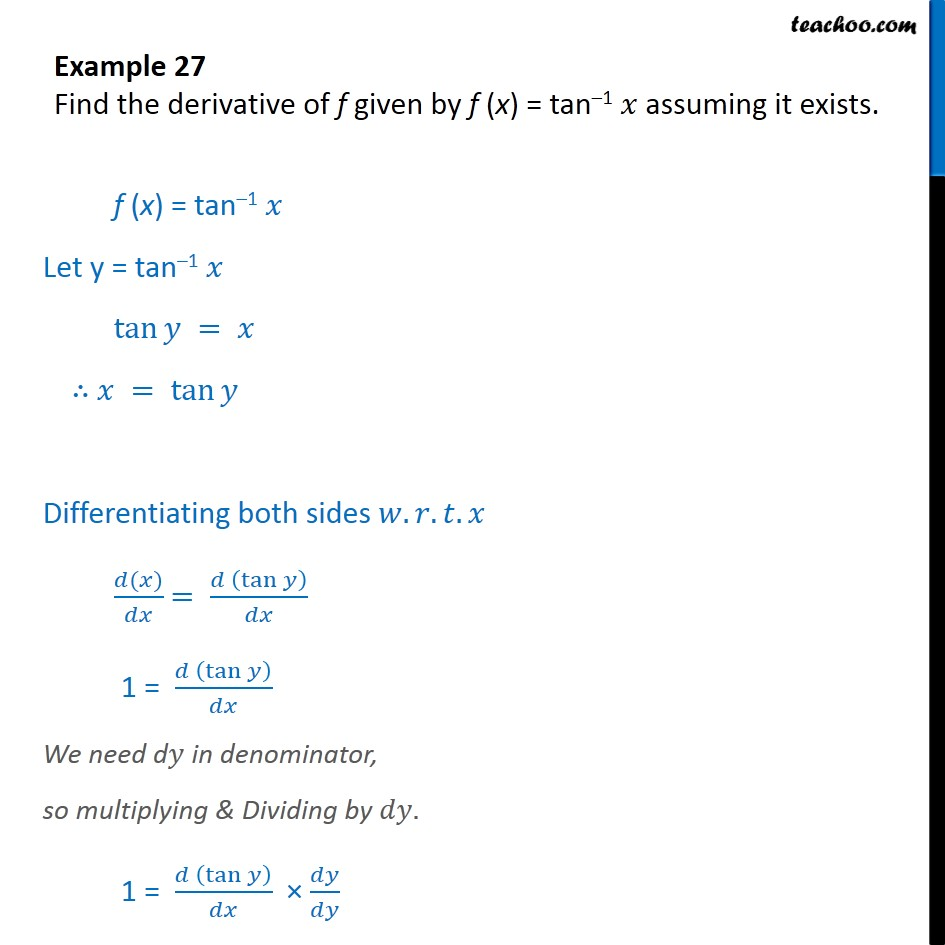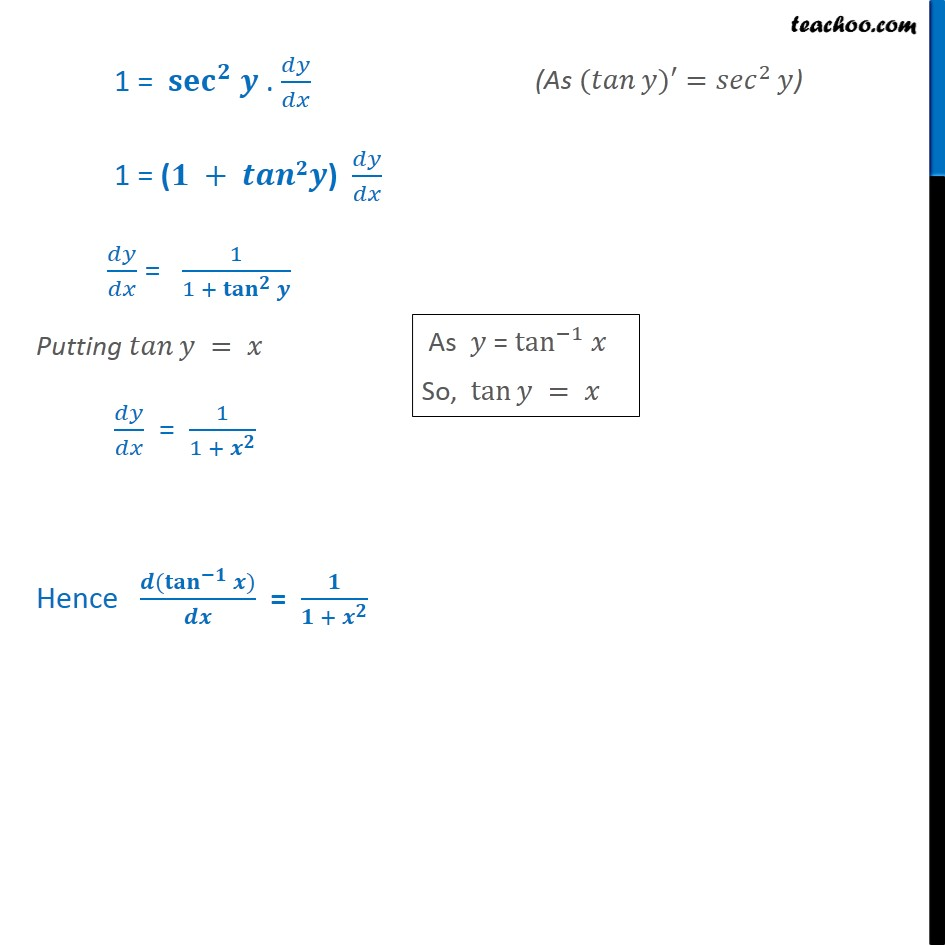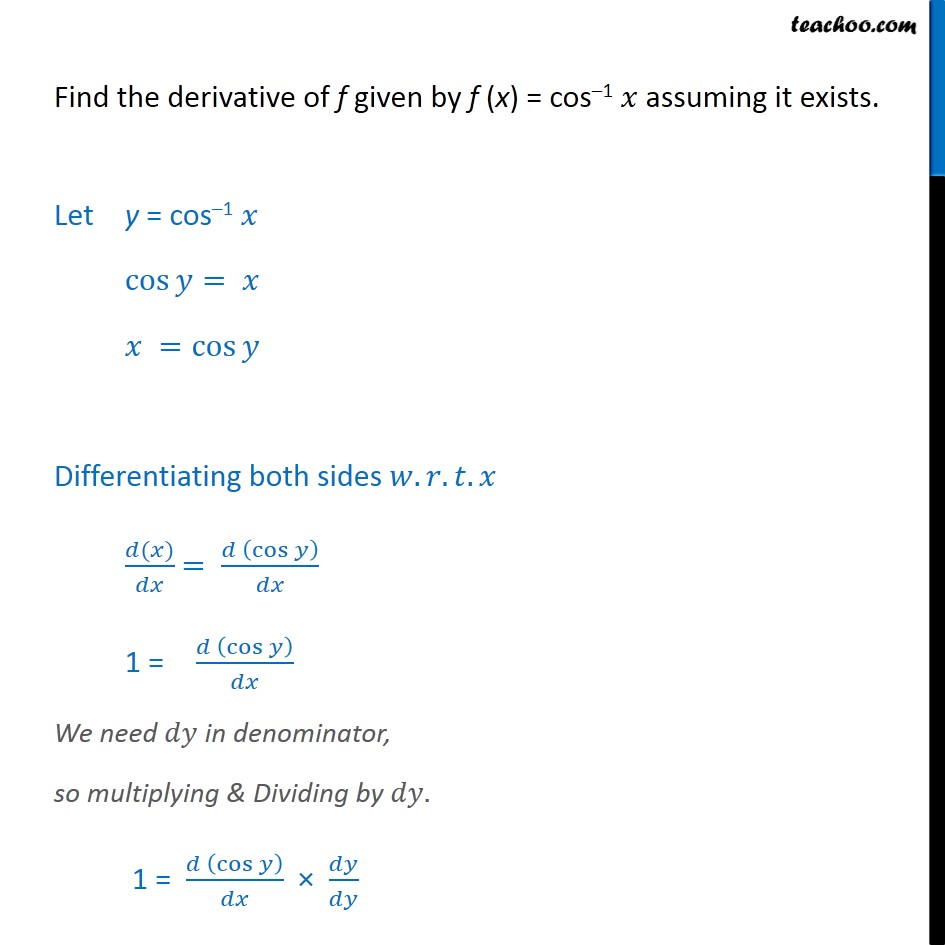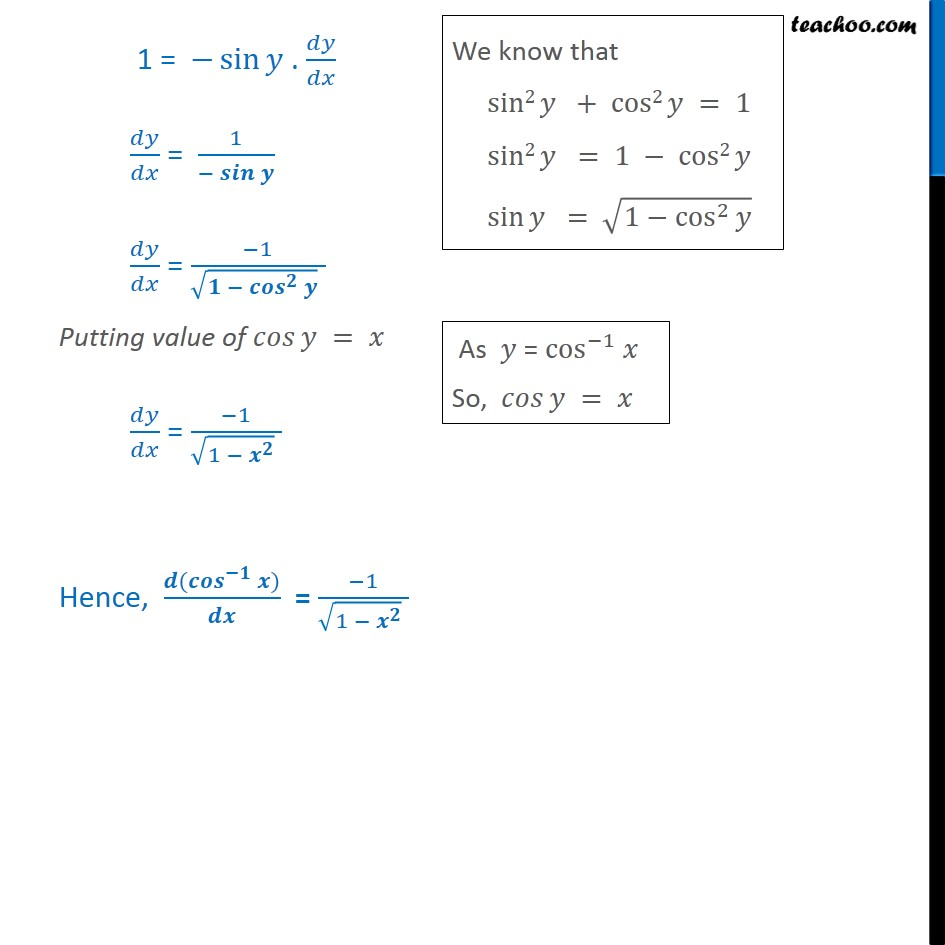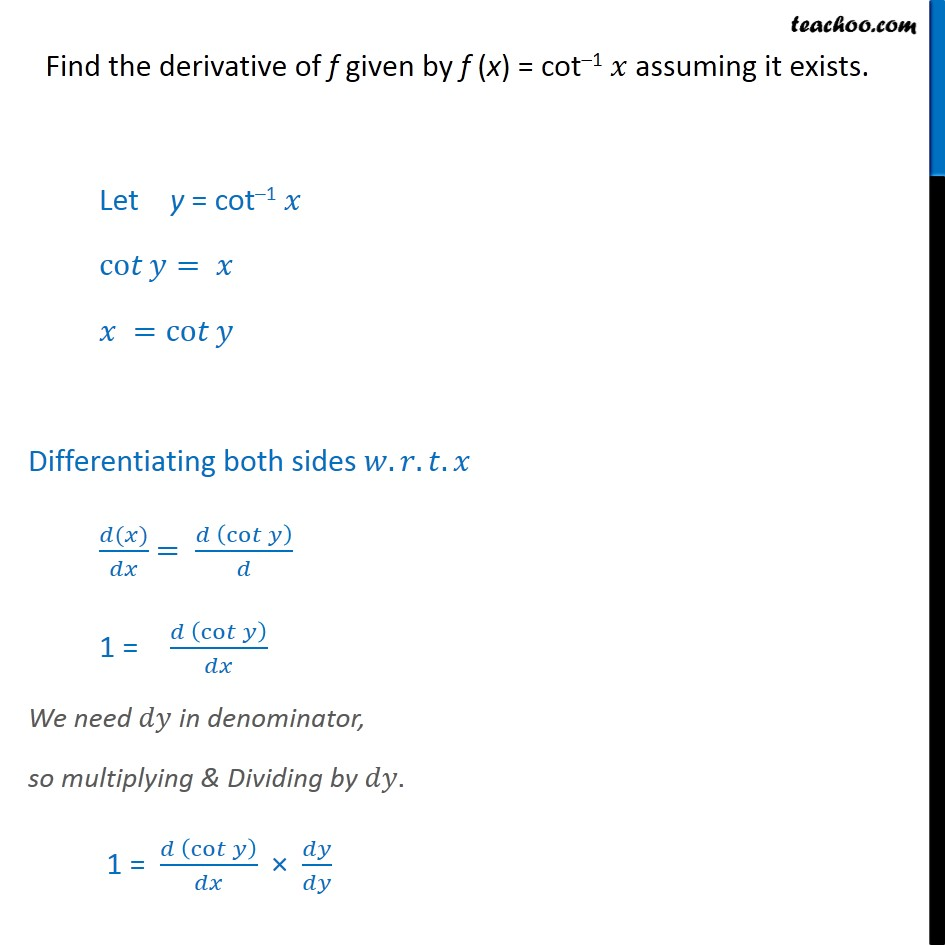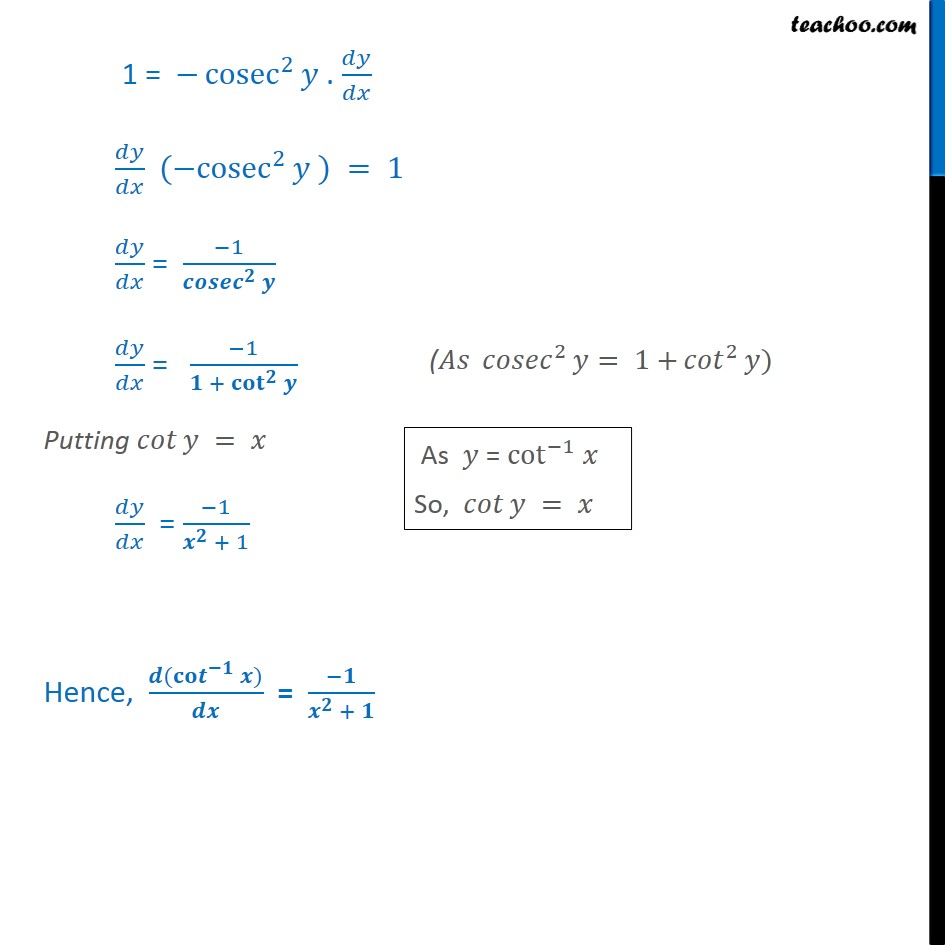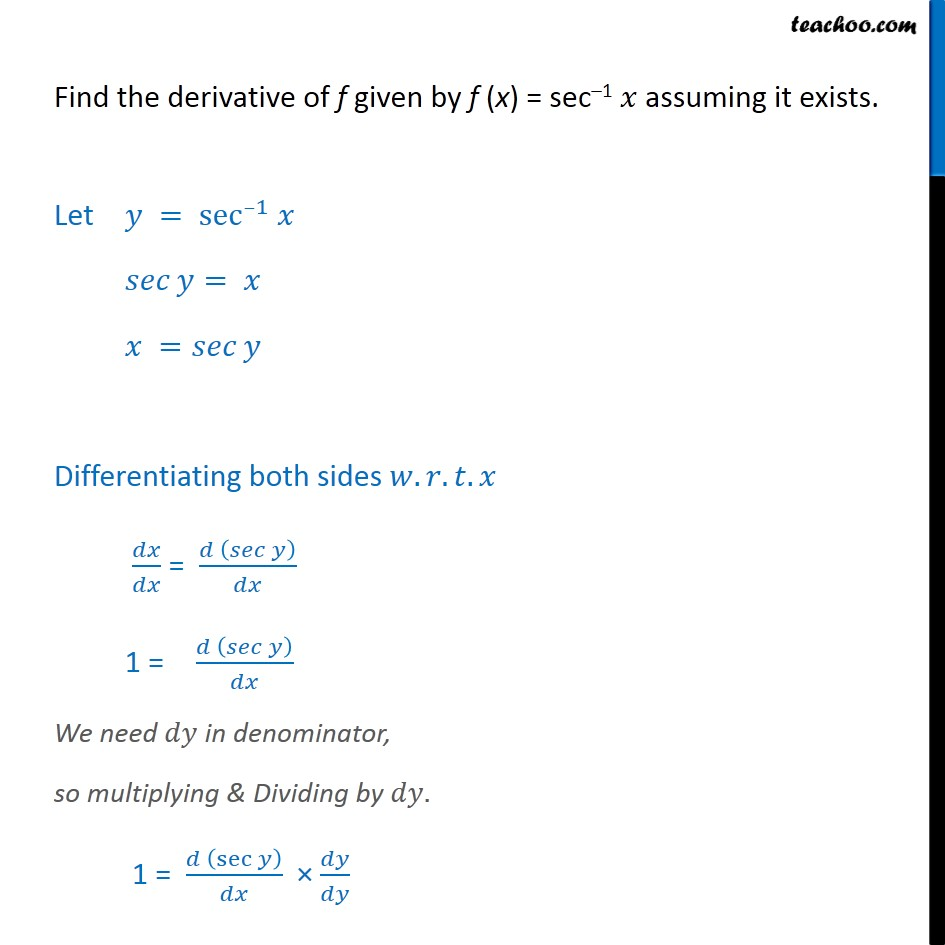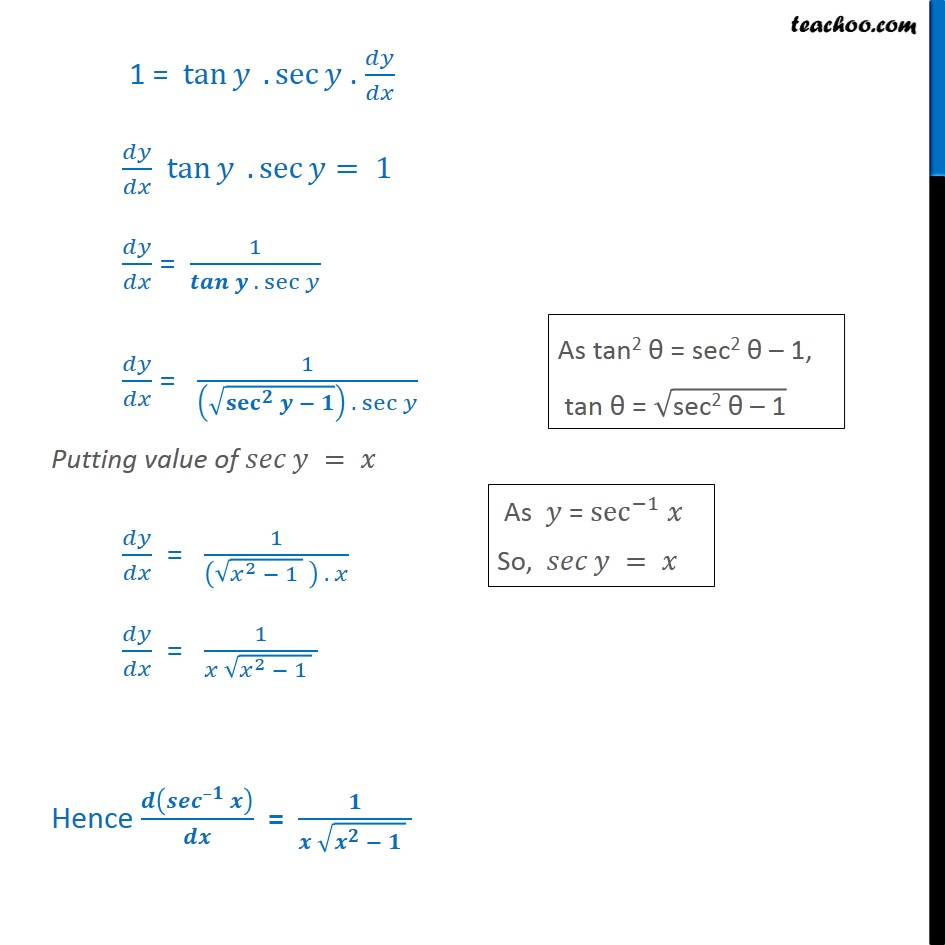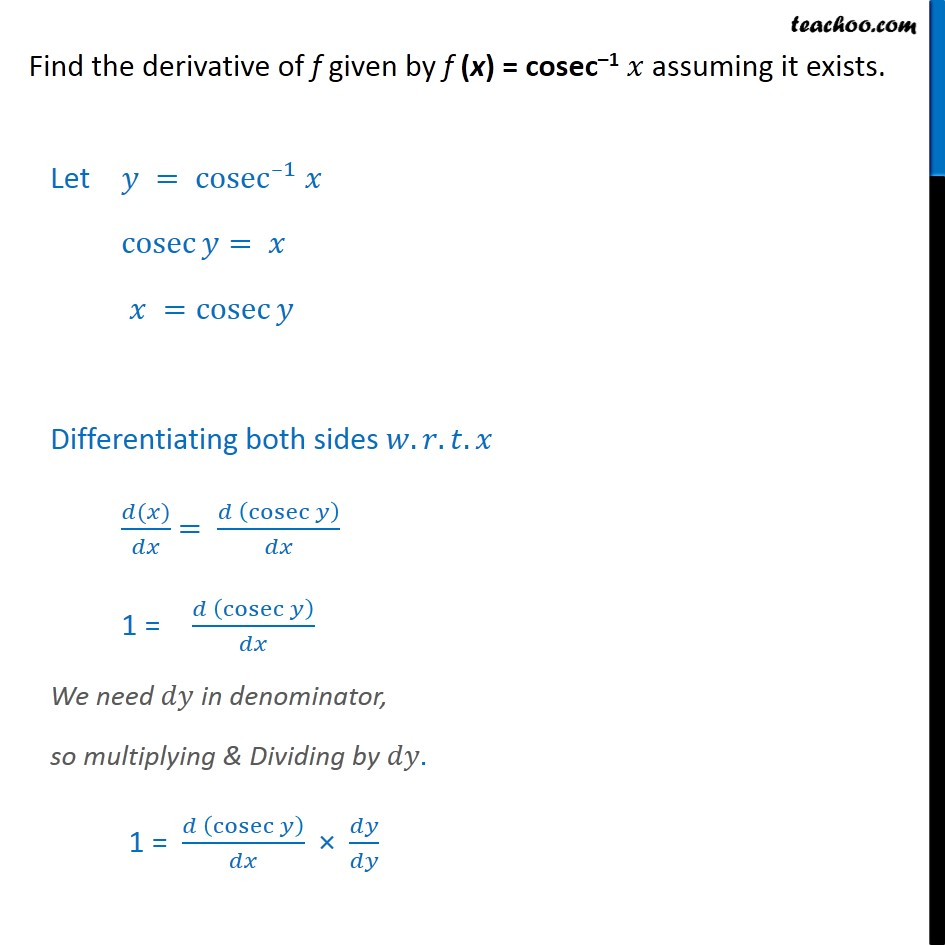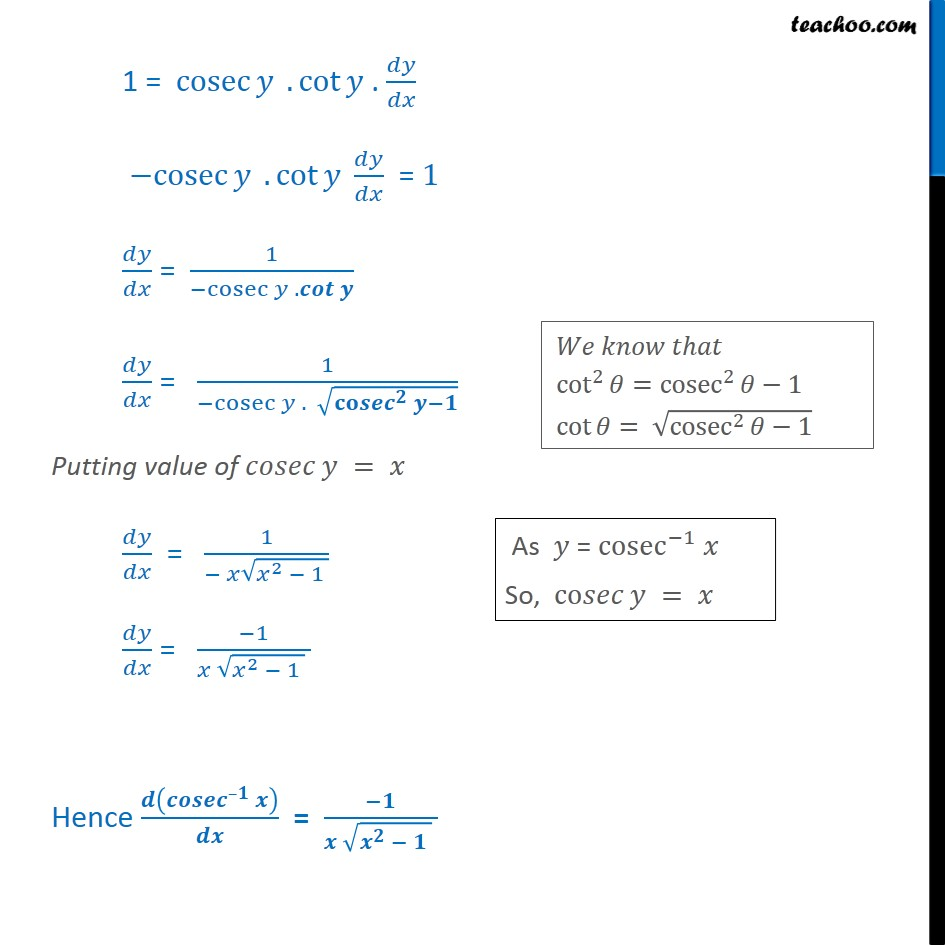1. Chapter 5 Class 12 Continuity and Differentiability
2. Serial order wise
3. Examples

Transcript

Example 27 Find the derivative of f given by f (x) = tan 1 assuming it exists. f (x) = tan 1 Let y = tan 1 tan = = tan Differentiating both sides . . . ( ) = tan 1 = tan We need d in denominator, so multiplying & Dividing by . 1 = tan 1 = . 1 = ( + ) = 1 1 + Putting = = 1 1 + Hence ( ) = + Find the derivative of f given by f (x) = cos 1 assuming it exists. Let y = cos 1 cos = = cos Differentiating both sides . . . ( ) = cos 1 = cos We need in denominator, so multiplying & Dividing by . 1 = cos 1 = sin . = 1 = 1 Putting value of = = 1 1 Hence, ( ) = 1 1 Find the derivative of f given by f (x) = cot 1 assuming it exists. Let y = cot 1 co = = co Differentiating both sides . . . ( ) = co 1 = co We need in denominator, so multiplying & Dividing by . 1 = co 1 = cosec 2 . ( cosec 2 ) = 1 = 1 = 1 + Putting = = 1 + 1 Hence, ( ) = + Find the derivative of f given by f (x) = sec 1 assuming it exists. Let = sec 1 = = Differentiating both sides . . . = 1 = We need in denominator, so multiplying & Dividing by . 1 = sec 1 = tan . sec . tan . sec = 1 = 1 . sec = 1 . sec Putting value of = = 1 2 1 . = 1 2 1 Hence = Find the derivative of f given by f (x) = cosec 1 assuming it exists. Let = cosec 1 cosec = = cosec Differentiating both sides . . . ( ) = cosec 1 = cosec We need in denominator, so multiplying & Dividing by . 1 = cosec 1 = cosec . cot . cosec . cot = 1 = 1 cosec . = 1 cosec . Putting value of = = 1 2 1 = 1 2 1 Hence =

Examples